Helmut
★★★Vienna, Austria,
2019-04-25 19:06

Posting: # 20235
Views: 1,124

“Average” plots [NCA / SHAM]

Dear all,

following this thread and esp. this post I hijacked Mittyri’s simulation code another time. As nobody rightly wrote

» Statistics is always some kind of abstraction.

Why are we using average* plots at all? The complete information is contained in spaghetti plots but Ohlbe remarked

» I find spaghetti plots rather difficult to read with more than 30 subjects, or even less depending on what they look like…

So which kind of average plot would allow a significant data reduction (one line per treatment) instead of one for every subject? Ideally it should convey unbiased information. I simulated 1,000 subjects and calculated averages as described there. It set the “LLOQ” of the method to 2% of the theoretical Cmax. If a concentration was calculated as BQL, I set it to NA. At the end I compared the averages with the theoretical concentrations at all time points i, i.e., calculated %REi = 100((xiCi) / Ci). Plotting %RE vs. t should give an idea which one to choose. I tried two flavors:
1. If the theoretical concentration C would be <LLOQ I forced it to NA.
2. I used it ‘as is’, which means that at 16 and 24 hours it is still used though it is far below the LLOQ.
Outcome:
1. All methods based on the arithmetic mean are terrible. Nothing new. The median where BQLs are imputed with LLOQ/2 seems to be the winner (always a moderate negative bias). Since the theoretical concentrations are BQL after 12 hours, no comparison possible.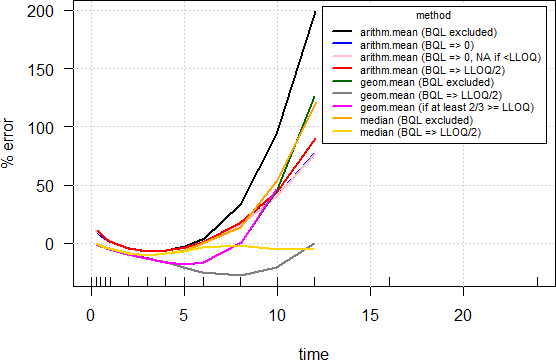2. Pretty similar but all go through the ceiling. Useless but gives an idea that we should take average curves in the terminal phase approaching the LLOQ with a grain of salt.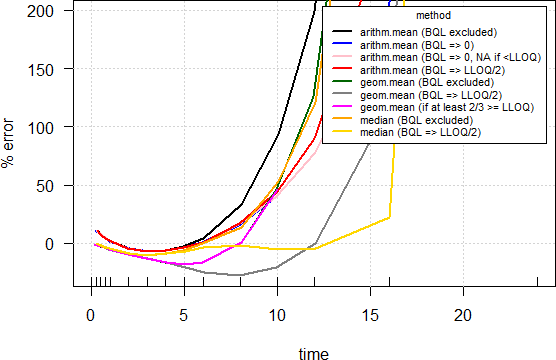• Average as a general term for a measure of location (mean, median, …).

R-code
C <- function(F=1, D, Vd, ka, ke, t) {
C <- F*D/Vd*(ka/(ka - ke))*(exp(-ke*t) - exp(-ka*t))
return(C)
}
loc.stat <- function(x, type, na.rm) {
non.numerics    <- which(is.na(suppressWarnings(as.numeric(x))))
x[non.numerics] <- NA
x <- as.numeric(x)
switch(type,
arith.mean = round(mean(x, na.rm=na.rm), 2),
median     = round(median(x, na.rm=na.rm), 2),
geom.mean  = round(exp(mean(log(x), na.rm=na.rm)), 2))
}
Nsub     <- 1000
D        <- 400
ka       <- 1.39
ka.omega <- 0.1
Vd       <- 1
Vd.omega <- 0.2
CL       <- 0.347
CL.omega <- 0.15
t        <- c(seq(0, 1, 0.25), seq(2,6,1), 8,10,12,16,24)
ke       <- CL/Vd
C.th     <- C(D=D, Vd=Vd, ka=ka, ke=ke, t=t)
tmax     <- log((ka/ke)/(ka - ke))
Cmax     <- C(D=D, Vd=Vd, ka=ka, ke=ke, t=tmax)
LLOQ.pct <- 2 # LLOQ = 2% of theoretical Cmax
LLOQ     <- Cmax*LLOQ.pct/100
df1      <- data.frame(t=t)
for (j in 1:Nsub) {
ka.sub <- ka * exp(rnorm(1, sd = sqrt(ka.omega)))
Vd.sub <- Vd * exp(rnorm(1, sd = sqrt(Vd.omega)))
CL.sub <- CL * exp(rnorm(1, sd = sqrt(CL.omega)))
df1    <- cbind(df1, C(D=D, Vd=Vd.sub, ka=ka.sub, ke=CL.sub/Vd.sub, t=t))
df1[which(df1[, j+1] < LLOQ), j+1] <- NA
}
names(df1)[2:(Nsub+1)] <- paste0("S.", 1:Nsub)
df2 <- data.frame(t(df1[-1]))
colnames(df2) <- df1[, 1] names(df2) <- t
loc <- data.frame(method=c("arithm.mean (BQL excluded)",
"arithm.mean (BQL => 0)",
"arithm.mean (BQL => 0, NA if <LLOQ)",
"arithm.mean (BQL => LLOQ/2)",
"geom.mean (BQL excluded)",
"geom.mean (BQL => LLOQ/2)",
"geom.mean (if at least 2/3 >= LLOQ)",
"median (BQL excluded)",
"median (BQL => LLOQ/2)"),
t.1=NA, t.2=NA, t.3=NA, t.4=NA, t.5=NA, t.6=NA, t.7=NA,
t.8=NA, t.9=NA, t.10=NA, t.11=NA, t.12=NA, t.13=NA, t.14=NA,
t.15=NA, stringsAsFactors=FALSE)
names(loc)[2:16] <- t
for (j in 2:(ncol(df2)+1)) {
x <- df2[, j-1]
loc[1, j] <- loc.stat(x, "arith.mean", TRUE)
y <- x
y[which(is.na(x))] <- 0
loc[2, j] <- loc.stat(y, "arith.mean", FALSE)
ifelse(loc[2, j] >= LLOQ, loc[3, j] <- loc[2, j], loc[3, j] <- NA)
y <- x
y[which(is.na(x))] <- LLOQ/2
loc[4, j] <- loc.stat(y, "arith.mean", FALSE)
loc[5, j] <- loc.stat(x, "geom.mean", TRUE)
loc[6, j] <- loc.stat(y, "geom.mean", FALSE)
if (length(which(!is.na(x))) >= nrow(df2)*2/3) {
loc[7, j] <- loc[5, j]
} else {
loc[7, j] <- NA
}
loc[8, j] <- loc.stat(x, "median", TRUE)
loc[9, j] <- loc.stat(y, "median", FALSE)
}
err2 <- err1 <- loc
C <- C.th
C[C.th < LLOQ] <- NA
for (j in 1:nrow(loc)) {
err1[j, 2:ncol(err1)] <- signif(100*(loc[j, 2:ncol(loc)]-C)/C, 4)
}
C <- C.th
for (j in 1:nrow(loc)) {
err2[j, 2:ncol(err2)] <- signif(100*(loc[j, 2:ncol(loc)]-C)/C, 4)
}
print(err1, row.names=FALSE);print(err2, row.names=FALSE)
clr <- c("black", "blue", "pink", "red", "darkgreen",
"gray50", "magenta", "orange", "gold")
dev.new(record=TRUE)
plot(x=t, y=rep(0, length(t)), type="n", xlim=range(t),
ylim=range(err1[, 2:16], na.rm=TRUE), xlab="time",
ylab="% error", las=1)
grid(); rug(t)
for (j in 1:nrow(err1)) {
lines(jitter(t, factor=1.5), err1[j, 2:ncol(err1)], col=clr[j], lwd=2)
}
legend("topright", bg="white", inset=0.02, title="method",
legend=loc[, 1], lwd=2, col=clr, cex=0.65)
plot(x=t, y=rep(0, length(t)), type="n", xlim=range(t),
ylim=range(err1[, 2:16], na.rm=TRUE), xlab="time",
ylab="% error", las=1)
grid(); rug(t)
for (j in 1:nrow(err2)) {
lines(jitter(t, factor=1.5), err2[j, 2:ncol(err2)], col=clr[j], lwd=2)
}
legend("topright", bg="white", inset=0.02, title="method",
legend=loc[, 1], lwd=2, col=clr, cex=0.65)

Cheers,
Helmut SchützThe quality of responses received is directly proportional to the quality of the question asked. ☼
Science Quotes
nobody
nothing

2019-04-25 20:17

@ Helmut
Posting: # 20237
Views: 1,025

“Average” plots

Median is always a robust parameter, as expected. Around LLOQ is always like twilight, be careful where you step...

Kindest regards, nobody
ElMaestro
★★★

Belgium?,
2019-04-25 22:53

@ Helmut
Posting: # 20238
Views: 1,000

“Average” plots

Hi Helmut,

» So which kind of average plot would allow a significant data reduction (one line per treatment) instead of one for every subject? Ideally it should convey unbiased information.

I am not using these plots for anything, and I do not know of anyone taken decisions on basis of them regardless of whether they involve means, medians, geomeans, with or without imputation, LLOQ/2 etc . For all I care, one flavour is as good as another and is of little importance.

I like simplifications, but reducing human PK profiles from e.g. 56 subjects to a single one in the hope that the result is particularly informative and/or allows decision-making is to me too ambitious.

Spaghetti plots can get confusing but all info is (potentially) in them. I don't like them particularly either.

One thing I'd like to try out, but I can't conjure up the code now:
Create a 95% (or 90% etc) shaded cloud around each mean or median at all time points. Now this results in two average/median/whatever profiles surrounded by its cloud, and the clouds will most often be overlapping (color shades can overlap in R). These sort of plots are sometimes used in ecological sciences and they have a fancy name over there. Like MacMillian-Hemorrhoid Gymnoplasticity Wexes or some such improbable term. My memory might not be entirely accurate here as to the nomenclature.
To me, this might be an improvement, but I can't say I'd use it for anything concrete until I get familiar with it.

I could be wrong, but...
Best regards,
ElMaestro
Helmut
★★★Vienna, Austria,
2019-04-26 01:08

@ ElMaestro
Posting: # 20239
Views: 1,000

Three shades of red

Hi ElMaestro,

» I am not using these plots for anything, and I do not know of anyone taken decisions on basis of them regardless of whether they involve means, medians, geomeans, with or without imputation, LLOQ/2 etc . For all I care, one flavour is as good as another and is of little importance.

True. On the other hand if I would dare to come up with a report without any my phone would not stop ringing…

» One thing I'd like to try out, but I can't conjure up the code now:
» Create a 95% (or 90% etc) shaded cloud around each mean or median at all time points.

Sumfink like those?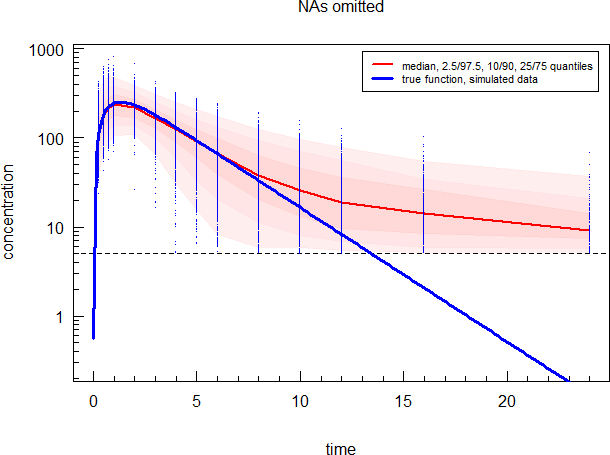Medians & quantiles, NA removed. LLOQ/2 imputation will be slightly closer to the true function. Too stupid to come up with confidence intervals of the truncated normal (for arithmetic means) or lognormal (for geometric means).

Interesting that the log-plot gives the false impression of a two-compartment model. Yep, there are lower concentrations at the end but we are not able to measure them.
Until now I knew it only the other way ’round: A true two-compartment model looks like a one-compartment model cause the analytical method is not sensitive enough.

Cheers,
Helmut SchützThe quality of responses received is directly proportional to the quality of the question asked. ☼
Science Quotes
nobody
nothing

2019-04-26 09:06

@ Helmut
Posting: # 20240
Views: 980

Three shades of red

I don't think that the problem with such plots is improper decision making based on such plots. It's that you might get the wrong impression from (a quick look at) the plot which prevents from asking the right questions... So: distraction instead of information.

Kindest regards, nobody
Helmut
★★★Vienna, Austria,
2019-04-26 18:27

@ nobody
Posting: # 20251
Views: 933

Three shades of red

Hi nobody,

» I don't think that the problem with such plots is improper decision making based on such plots. It's that you might get the wrong impression from (a quick look at) the plot which prevents from asking the right questions... So: distraction instead of information.

See what Detlew wrote about lies. Now I understand why it is possible in Phoenix/WinNonlin to define two rules to deal with BQLs and/or missings. One for NCA (the real stuff) and another one for plotting.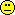Couldn’t resist: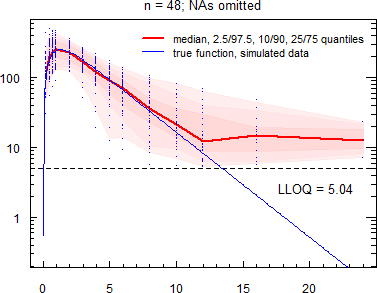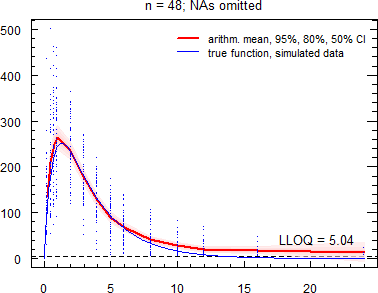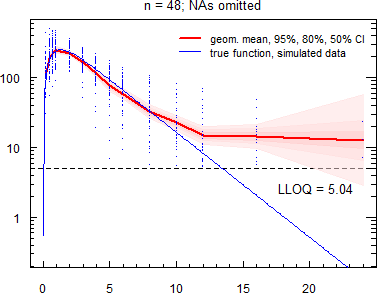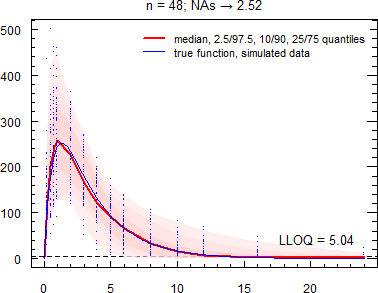Cheers,
Helmut SchützThe quality of responses received is directly proportional to the quality of the question asked. ☼
Science Quotes
ElMaestro
★★★

Belgium?,
2019-04-26 09:10

@ Helmut
Posting: # 20241
Views: 977

Three shades of red

Hi Hötzi,

» Sumfink like those?

Yes, that's approximately it!!! With gymnoplastic compliments, I have to say "well done" to youI could be wrong, but...
Best regards,
ElMaestro
Bioequivalence and Bioavailability Forum |  Admin contact
19,889 posts in 4,215 threads, 1,364 registered users;
online 6 (0 registered, 6 guests [including 3 identified bots]).
Forum time (Europe/Vienna): 08:16 CEST

I have no opinion about ‘incurred samples’ –
an expression which has no easily understandable
meaning for me in the English language.    Nick Holford

The BIOEQUIVALENCE / BIOAVAILABILITY FORUM is hosted byIng. Helmut Schütz